# Module Coupling Slice – based Test case Prioritization

Module-coupling slice-based test case prioritization is a technique that uses the module-coupling value in order to identify the critical modules in the software and hence prioritize the test cases in the test suite based on these critical modules.

Since most of the test case prioritization techniques do not take into account the fact that change in one module could also be propagated in other modules of the software. So, instead of prioritizing test cases on a simple criterion like coverage, risk, requirements, etc., the critical modules could be identified based on which test case prioritization could be performed. Below is the algorithm used for performing module-coupling slice-based test case prioritization:

#### Algorithm for the Module-coupling slice-based test case prioritization

1. Firstly, we create a module coupling matrix C which indicates the coupling among all the modules in the program. Construct an m X m module coupling matrix, where m is the number of modules in the program. Fill the matrix using the module-coupling values, i.e. Cij represents the coupling between module i and module j. The module coupling matrix is symmetric in nature, i.e. Cij = Cji, and all the diagonal elements i.e. Cii for all is equal to 1.
2. Construct a module cohesion matrix S by using the cohesion type of each module and the corresponding value for each cohesion type. This is a 1 X m matrix, where m is the number of modules in the program.
3. The final step is to create a module dependency matrix D, which is an m X m matrix, where m is the number of modules in the program. It is constructed using the following equation:
```Dij = 0.15 * ( Si + Sj ) + 0.7 * Cij

where, Cij is not equal to 0
Also,
Dij = 0 where Cij = 0
Dii = 1 for all i

```

As we can see, the module coupling matrix C and module cohesion matrix S is used to construct the module dependence matrix D. The link having the highest value in the module dependence matrix are given the highest priority and the test cases are prioritized for the same modules that are associated with those links.

#### Solved Example

Question: Using the following call graph and tables, construct the Module Coupling, Module Cohesion, and Module Dependency Matrices: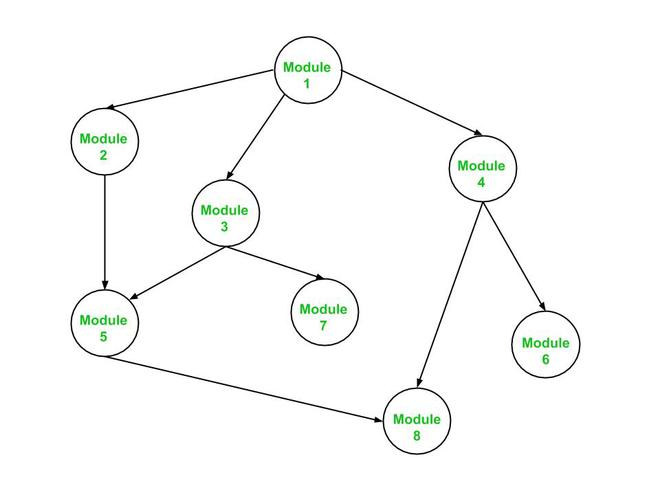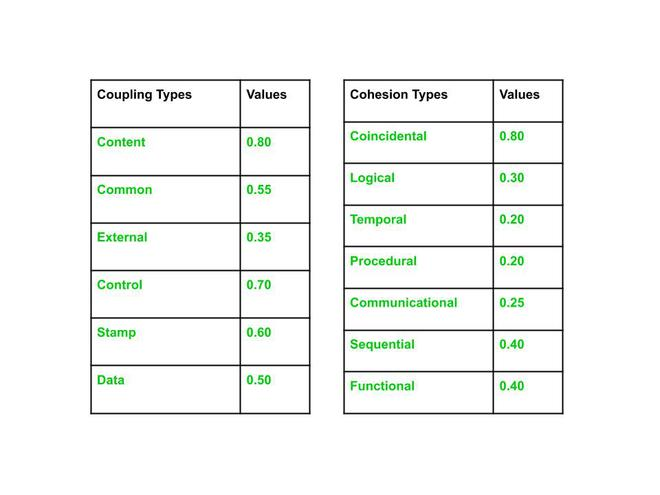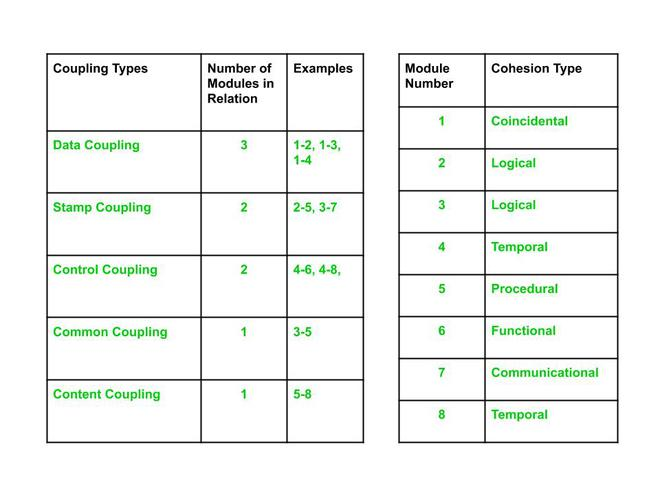Solution

Using the Algorithm stated above, we can compute the matrices as follows:

1. Module Coupling Matrix (C)

Create an m x m  matrix, where m is the number of modules. Here, from the given call graph we can see that there are eight modules and hence the value of m = 8. So, create a 8 x 8 matrix and start filling it on the basis of the value that is associated with the coupling type between modules i and j

```Example 1:

For the link 1-2 in call graph,
the coupling type = Data Coupling (Refer given table in the Question)
i = 1 and j = 2
C12  =  0.50 since value associated with Data Coupling Type is 0.50

Example 2:

For the link 3-7 in call graph,
the coupling type = Stamp Coupling (Refer given table in the Question)
i = 3 and j = 7
C37 = 0.60 since value associated with Stamp Coupling Type is 0.60
```

Fill the entire matrix in a similar manner and the complete Module Coupling Matrix will be as shown below: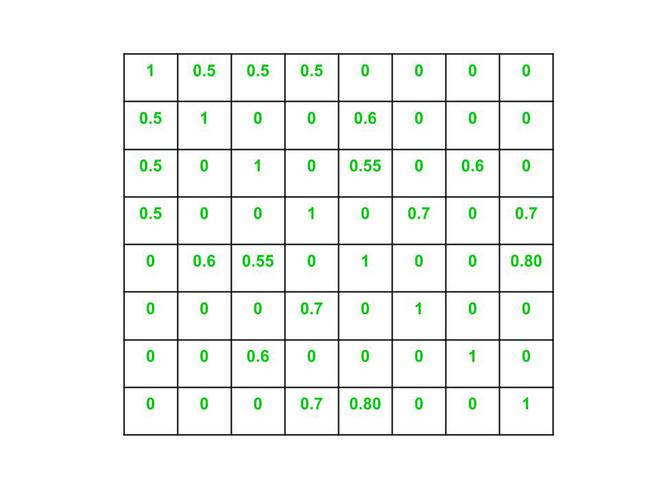2. Module Cohesion Matrix (S)

It is a 1 x m matrix which is simple to create using the tables provided in the question. Use the module cohesion type and the values associated with the cohesion types to fill this matrix.

```Example 1:

For the module 1 in call graph,
the cohesion type = Coincidental (Refer given table in the Question)
S1 = 0.80 since value associated with Coincidental Cohesion Type is 0.80

Example 2:

For the module 4 in call graph,
the cohesion type = Temporal (Refer given table in the Question)
S4 = 0.20 since value associated with Temporal Cohesion Type is 0.20
```

Fill the entire cohesion matrix as described above and the complete Module Cohesion Matrix will be: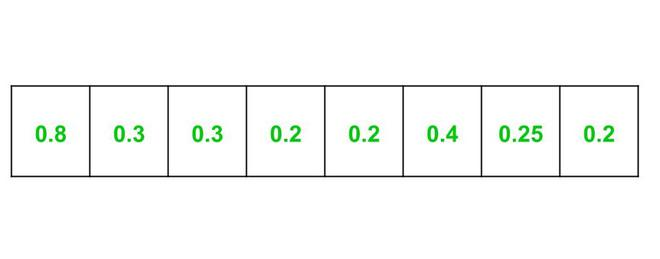3. Module Dependency Matrix (D)

It is an m X m matrix that is constructed using both Module Coupling and Module Cohesion Matrix. Use the formula stated in the algorithm.

```Example 1:

For the link 1-2 in call graph,
D12 = 0.15 * ( S1 + S2 ) + 0.7 * C12
Since S1 = 0.80, S2 = 0.30 and C12 = 0.50
Therefore,
D12 = 0.15 * ( 0.80 + 0.30 ) + 0.7 * 0.50
= 0.515 = 0.52 (round off to 2 decimal places)

Example 2:

For the link 4-6 in call graph,
D46 = 0.15 * ( S4 + S6 ) + 0.7 * C46
Since S4 = 0.20, S6 = 0.40 and C46 = 0.70
Therefore,
D46 = 0.15 * ( 0.20 + 0.40 ) + 0.7 * 0.70
= 0.58
```

After filling the entire matrix, the module dependence matrix will be: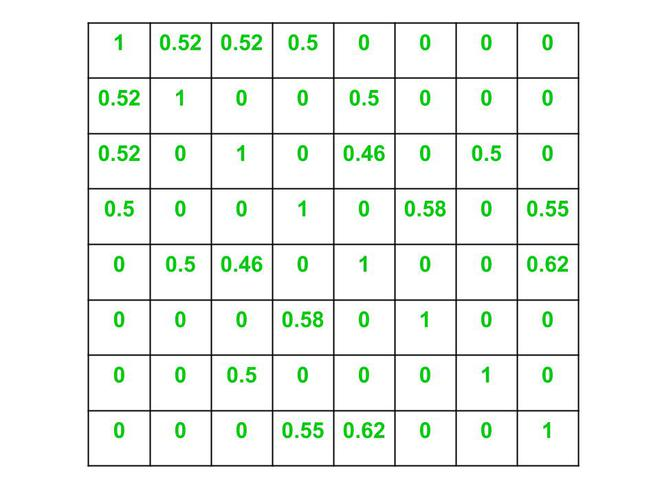From the Module Dependency Matrix shown above, we can see that modules 4, 5, 6, and 8 have the highest value in the dependence matrix obtained, and hence test cases for these modules should be given higher priority as compared to other modules.

Attention reader! Don’t stop learning now. Get hold of all the important CS Theory concepts for SDE interviews with the CS Theory Course at a student-friendly price and become industry ready.

My Personal Notes arrow_drop_upCheck out this Author's contributed articles.

If you like GeeksforGeeks and would like to contribute, you can also write an article using contribute.geeksforgeeks.org or mail your article to contribute@geeksforgeeks.org. See your article appearing on the GeeksforGeeks main page and help other Geeks.

Please Improve this article if you find anything incorrect by clicking on the "Improve Article" button below.